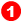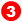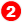﻿ Dialog: Function-Block: Gearing

# Dialog: Function-Block: Gearing

## Gearing FB

### Gearing FB

The Gearing FB is a linear equation with three arguments / parameters:

Output = (a × (input + b)) + c

'a'  - enter an Irrational Number, or a Ratio of two numbers.

### Edit the Gearing FBEdit the Gearing FB:

 1 Double-click the Gearing FB in the graphic-area.

- or -

 1

### Gearing FB dialog-box

Default output:

output motion-value = input motion-value

Output Function:

Three parameters:

 The Gearing FB function is: Output  = {×(Input +)}+Gearing FB dialog-box

Ratio and PhaseGearing Ratio [See also - below Gearing as 'Real' or 'Ratio' ]Add after Gear RatioAdd before Gear Ratio

Gearing as 'Real' or 'Ratio'Gearing Ratio:

 ◉ Gearing as 'Real' - enter any number in the Gearing Ratio- or -

 ◉ Gearing as 'Ratio' - enter integers in&Gearing Ratio= Input-Pulley / Output-PulleyInput-Pulley = ± IntegerOutput-Pulley = ± Integer

Notes:

Gearing Ratio:

-1 > Gearing Ratio > 1 the output is 'stepped up', and becomes greater in magnitude than the input

-1 < Gearing Ratio < 1 ; the output is 'stepped down', and becomes less in magnitude than the input

Gearing Ratio < 0 ; the output is opposite to [negative of] the input.

Gearing Ratio = 1 ; the output is equal to the input

Gearing Ratio =-1 ; the output is equal, but the opposite to [negative of] the input

Connect a Gearing FB to the input of a Motion FB to change the number of times you complete a Motion in one Machine Cycle - Motion Frequency

Connect the output of a Motion FB to the input of a Gearing FB to change the amplitude of the motion - Motion Amplitude/Range.